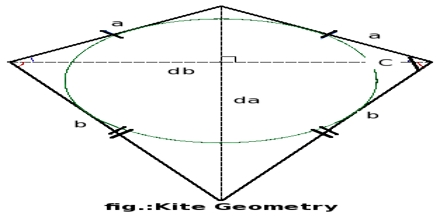Mathematic

# What are Kites?Kite is a quadrilateral that has two pairs of consecutive congruent sides, but opposite sides are not congruent. Kites were first developed in ancient China. This lecture briefly describe on properties of kites: the diagonals of a kite are perpendicular, non-vertex angles (a)are congruent, vertex diagonal bisects the vertex angles and Vertex diagonal bisects the non-vertex diagonal.